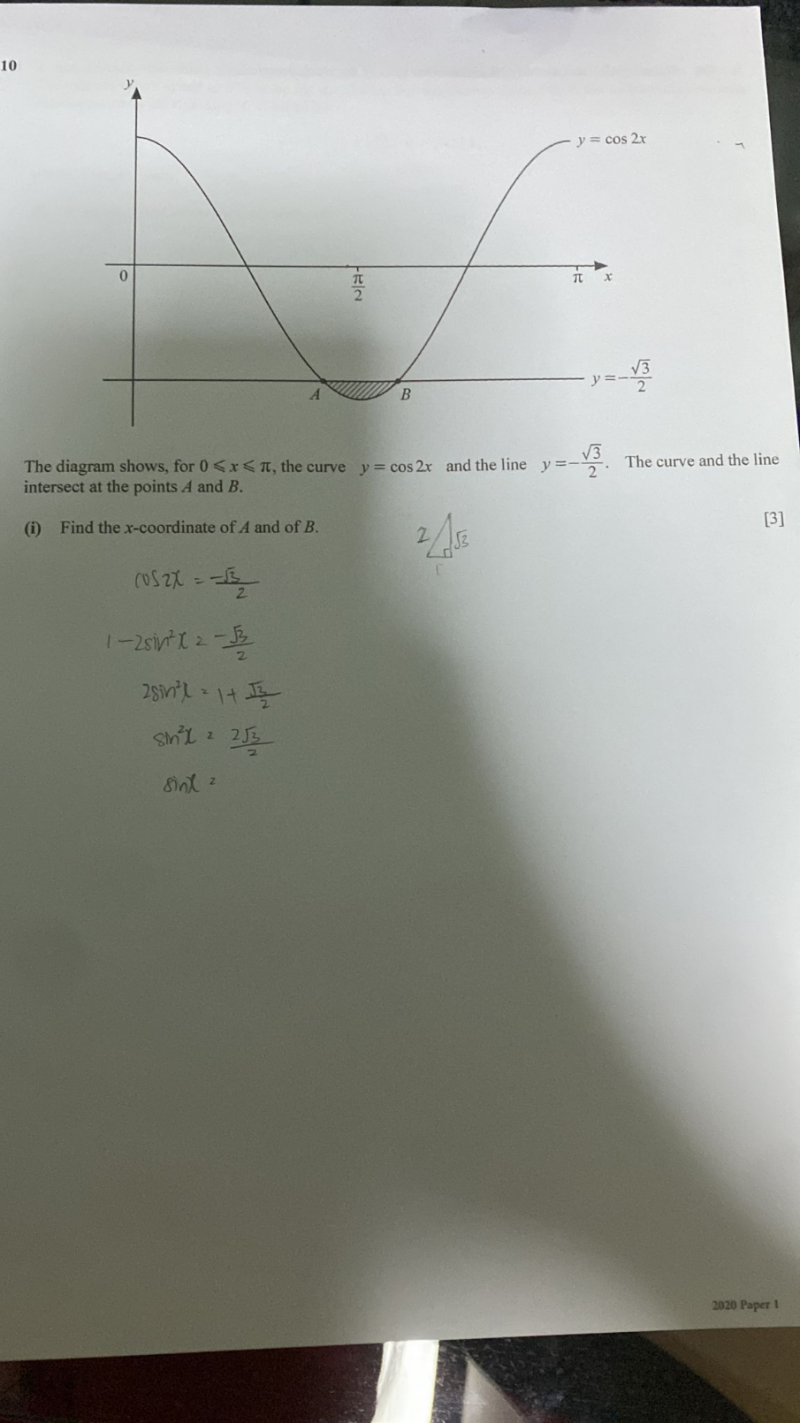# QuestionHow to continue thank you

cos 2x -(3)/2

We know cos (π/6) = (3)/and cos ( π – P ) =-cos P

cos (π – (π/6)) = – cos (π/6)

cos ((5π)/6) = – (3)/2

2x = (5π)/6   => x = (5π)/12     y = -(3)/2         for         0 < x < π

Hence coordinate of A = ( (5π)/12 , -(3)/2 )

We also know cos (π/6) = (3)/and cos ( π + P ) =-cos P

cos (π + (π/6)) = – cos (π/6)

cos ((7π)/6) = – (3)/2

2x = (7π)/6   => x = (7π)/12     y = -(3)/2         for         0 < x < π

Hence coordinate of B = ( (7π)/12 , -(3)/2 )

0 Replies 2 Likes ✔Accepted Answer